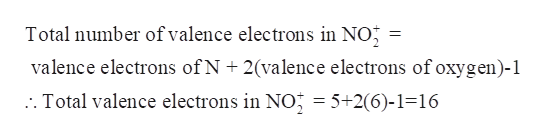Question
13 views

How do you draw Lewis Dot Structures?

check_circle

Step 1

Lewis Dot structure-Lewis structures are the diagrams that show how the atoms are covalently bonded in a molecule and also show the arrangement of lone pair of electrons around the atoms.

Step 2

Steps to draw a Lewis Dot structure-

1. Select the central atom- usually the least electronegative atom is chosen as the central atom. For example in CO2, Carbon is the central atom.
2. Determine the total number of valence electrons in the molecule- Total number of electrons in a molecule is calculated by adding the valence electrons of each atom.

For ions, add or subtract the number of electrons necessary to achieve the total charge.

1. Place a bonding pair of electrons between atoms to show the connectivity of the atoms.
2. Now, place the electrons around each atom in order to complete its octet (two for hydrogen) - these electrons are placed as lone pairs around the atoms. Begin this with the terminal atoms followed by the central atom.
3. If the octet of central atom is not complete, then try forming double bonds with the other atoms and adjust the lone pairs of terminal atoms as per the new structure.
4. Total number of valence electrons remains the same.
Step 3

Example – nitronium ion (NO2+)

Nitrogen is less electronegativ...help_outlineImage TranscriptioncloseTotal number of valence electrons in NO valence electrons of N + 2(valence electrons of oxygen)-1 . Total valence electrons in NOj = 5+2(6)-1-16 fullscreen

### Want to see the full answer?

See Solution

#### Want to see this answer and more?

Solutions are written by subject experts who are available 24/7. Questions are typically answered within 1 hour.*

See Solution
*Response times may vary by subject and question.
Tagged in

### Inorganic Chemistry